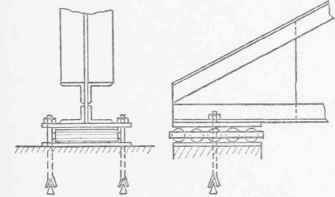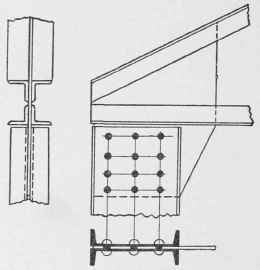If a truss rests on masonry walls, three methods of making the details at L0 are in common use. These are shown in Figs. 55, 56, and 57. The detail shown in Fig. 55 is the most commonly used; but its use is not advised unless a sufficient number of rivets are placed in the members to take up both the direct stress and that due to the fact that the point of application of the reaction does not coincide with the intersection of the center lines of the chord members.Fig. 52. Slotted-Hole Expansion Bearing.Fig. 53. Roller Expansion Bearing.Fig. 51. Rocker Expansion Bearing.Fig. 55.Fig. 56.Fig. 57. Details of Ends of Roof Trusses.

In case the bearing shown in Fig. 55 is used, the number of rivets in L0 L1 may be calculated from the equation: n2v - Rn = 6 Re/ p, in which, n = Number of rivets required; v = Allowable stress on one rivet; R - Vertical reaction; p = Rivet spacing, in inches; e = Distance as shown in Fig. 55.

If the number of rivets in L0 Ul is desired, it may be calculated from the equation: n-v - Sn = 6 Se1 /P in which S is the stress in L0 U1 e the distance shown in Fig. 55, and the remaining notation as above.

If the point of application of the reaction coincides with the intersection of the center lines of the top and bottom chords, the number of rivets required to withstand the direct stress, which is the only stress would be equal to the stress in that member divided by the allowable stress in one rivet.FRONT VIEW OF HOUSE AT ST. PAUL, MINN.

John T. Comes, Architect, Pittsburg, Pa.

Cost of House, \$7,500. For Plans, See Page 266.REAR VIEW OF HOUSE AT ST. PAUL, MINN.

John T. Comes, Architect, Pittsburg, Pa.

For Plans, See Page 266.

In order to illustrate the use of the above equation, and to bring out the fact that more rivets are required when the point of application of the reaction does not coincide with the intersection of the upper and lower chords than when it does coincide, an example will be solved. The stresses, the thickness of the connection plate, and the distance of the point of application of the reaction from the intersection of the chord, are as shown in Fig. 58.

It will be assumed that the chords consist of two angles each; and since this is the case, the allowable unit-stress in one rivet will beFig. 58. Data for Example on Page 37.Fig. 59. Eave Detail for Fig. 57.

3 750 pounds, the value of a ¾-inch rivet in bearing in a ¼-inch plate when the allowable unit bearing stress is 20 000 pounds per square inch. If the point of application of the reaction coincides with the intersection of the two chords, the number of rivets required will be:

For L0 U1 45 000 / 3 750 = 12.00 rivets.

For L0 L1 40 000 /3 750 = 10.67 rivets.

Since the point of application of the reaction does not coincide with the intersection of the chord, the number of rivets required in L0Ul is:

3 750 n2 - 45 000 n = 6 X 45 000 X4.44 / 3 , the spacing being 3 inches; dividing by 3 750, we have: n2 - I2n = 106. 56.

Completing the square and solving for n, there is obtained: n = 6 += 17.9, say 18 rivets.

The number of rivets required in L0 L1 is:

3 750 n2 - 20 000 n = 6 X 20 000 X 10 / 3;Fig. 60.Fig. 61.Fig. 62.Fig. 63. Details of Tops of Columns.

and dividing by 3 750 and completing the square, there results: n = 2.67 +=13.32, say 14 rivets.

Inspection of the above results shows that when the point of application of the reaction is placed 10 inches from the intersection of the chords, it requires 6 more rivets in the upper chord and 3 more rivets in the lower chord than would be required if the point of application of the reaction coincided with the intersection of the chords.

The detail just discussed is a very convenient one, and is very commonly used; but in most cases no allowance is made for the additional rivets required because of the fact that the reaction does not coincide with the intersection of the chord members. The student should always compute the rivets by the formulae given above, since it is very evident that neglect to do so causes the joint to be exceedingly weak, in some cases as much as 50 per cent, as is shown in the case of L0 Ll in the above problem.

Fig. 56 is excellent, but the length of the bearing plate, which should be as long as the connection plate, is liable to become greater than the width of the wall. In such cases the detail shown in Fig. 57 is to be used. The objection raised to these details is that the end connection plate prevents the placing of a purlin near the end of the roof truss. In case sheathing is used, this objection does not hold good, since the overhanging sheathing will reach to the end of the truss and form a good eave detail, as shown in Fig. 50.